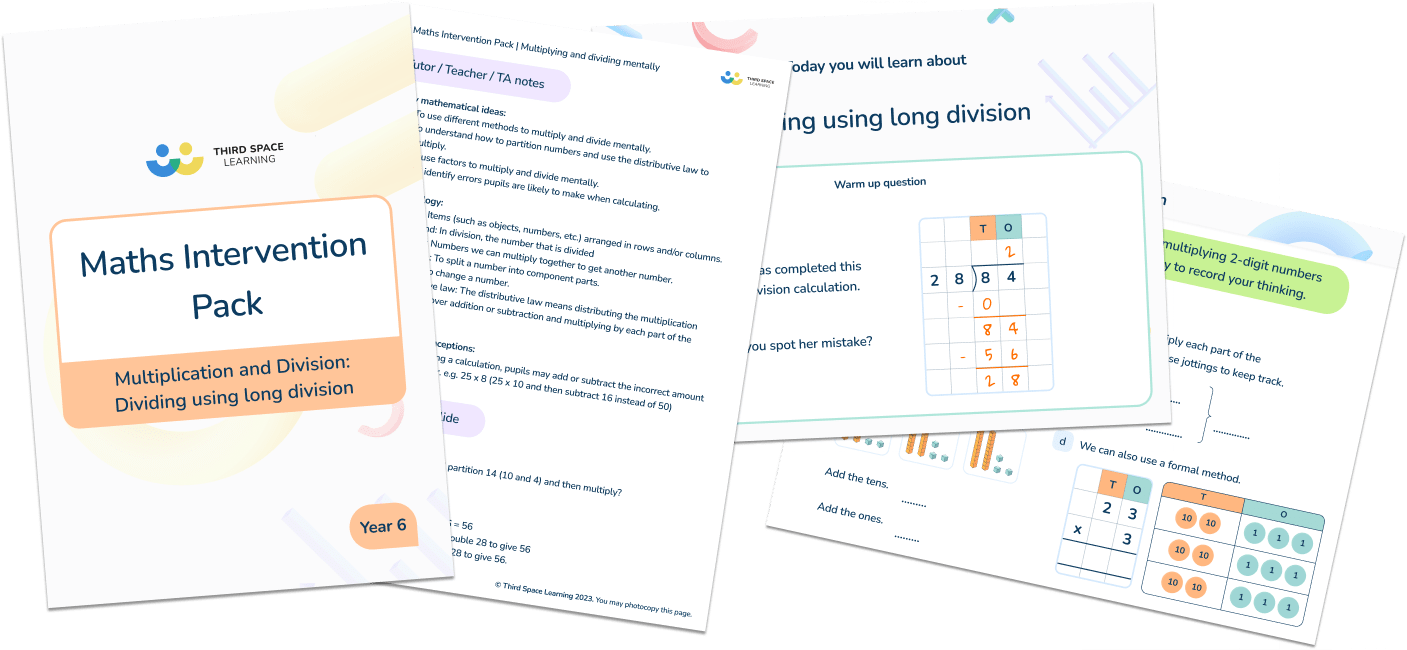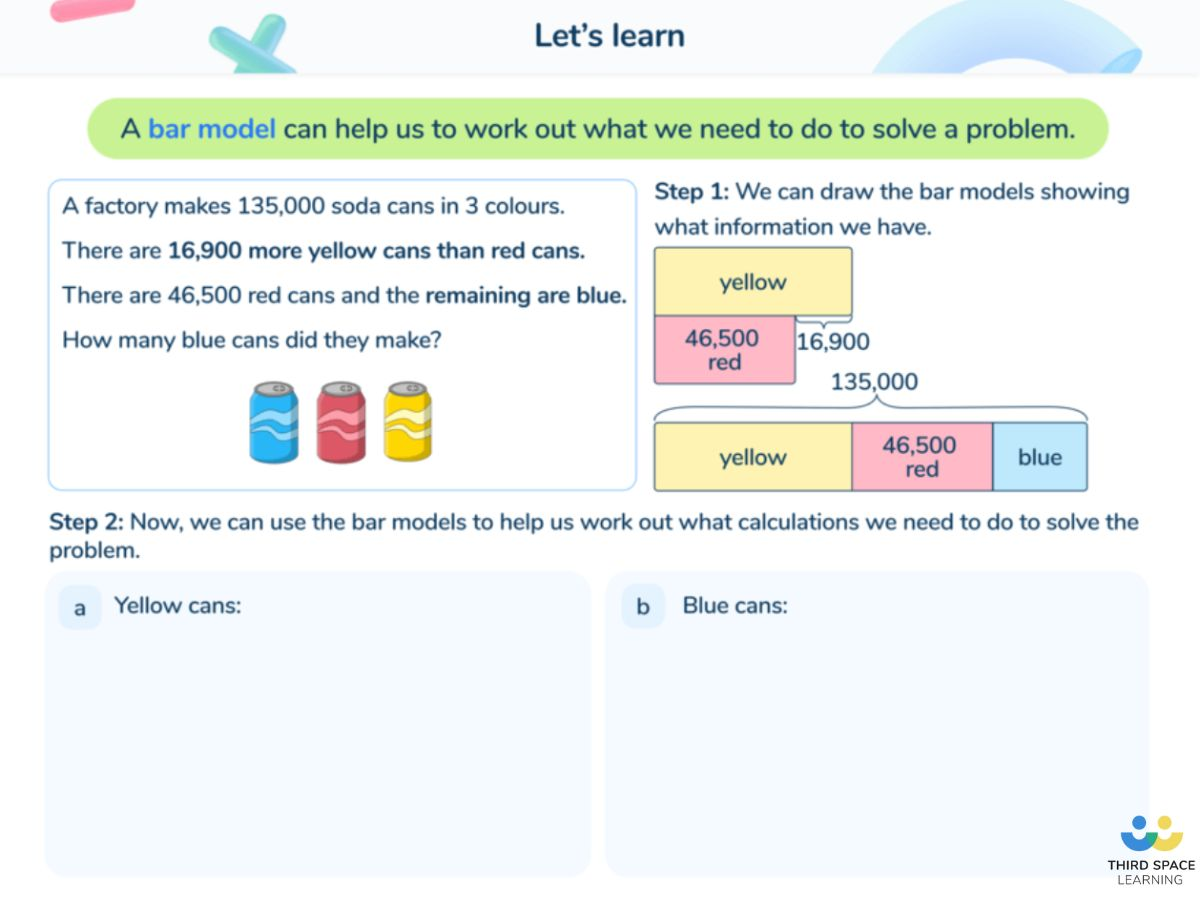Teaching support from the UK’s largest provider of in-school maths tuitionone to one lessonsschools supported

Built by teachers for teachers

In-school online one to one maths tuition developed by maths teachers and pedagogy experts

Find out more

Hundreds of FREE online maths resources!

Daily activities, ready-to-go lesson slides, SATs revision packs, video CPD and more!Explore all resources
Maths Mastery

Built by teachers for teachers

In-school online one to one maths tuition developed by maths teachers and pedagogy experts

Find out more

# Bar Model Multiplication And Division: How To Teach Multiplication And Division Using The Bar Model

Bar model multiplication is an effective method for teaching multiplication at KS1 and KS2. As student’s progress towards their times table check in Year 4, it’s important to instil a deep understanding of the concept of multiplication as repeated addition, rather than rote memorisation of multiplication tables: bar model multiplication is a great way to do this.Maths Intervention Pack Multiplication and DivisionDownload Free Now!

### What is bar model multiplication and division?

What is a bar model? Bar modelling is a maths mastery technique and part of the Singapore math framework. Bar model multiplication and division utilises the bar model method, pictorial representation and the concrete pictorial abstract approach to illustrate the concept of multiplication and division.

Bar models can also be used to teach maths concepts such as fractions, using a fraction bar model, percentages, place value, decimals, addition, subtraction and can be helpful for solving word problems.

### Why use bar model multiplication and division

Children begin learning simple multiplication and division in early years. The concept of multiplication is typically introduced by adding the same number together multiple times. By using a bar model, teachers can demonstrate that one whole can be divided into equal, smaller parts.

Teachers can draw bar models to demonstrate this:

By drawing a bar model and separating the bar into equal parts, children can visualise the process of multiplication and division and how they have an inverse relationship.

### Bar models and problem solving

Bar models are particularly useful when it comes to solving one-step multiplication word problems, two-step maths problems and multi-step word problems. Bar models enable students to extract the most important information to solve number problems.

Third Space Learning often incorporates bar modelling into our online one-to-one tuition lessons. Paying close attention to each individual student,Third Space Learning identifies their learning gaps and moulds the programme to their needs.Third Space Learning’s online one to one tutoring sessions incorporate different strategies to support student problem solving, including bar models.

Read more: Year 6 word problems

#### Bar model multiplication in action

Here is an example of a multiplication word problem and how it can be solved using bar model multiplication:

Mary has 26 flowers, John has 3 times the amount of flowers as Mary and Jane has 14 more flowers than John. How many flowers does Jane have?

In this example, the bar model is helpful in identifying the missing number. By presenting the problem pictorially, pupils can more easily understand the steps they need to take to solve the problem.

#### Example of bar model division

Here is an example of a division word problem and how it can be solved using a bar model.

Tony spends 56 hours working each week. How many hours a day on average is Tony working?

This kind of part whole model depiction can help students understand what division really means by demonstrating that division is the act of breaking up numbers into equal groups.

### More multiplication & division and bar models resources

Third Space Learning offers a large collection of learning resources including lesson plans, worksheets and online one-to-one tuition. Visit our maths hub to view our library of popular primary maths resources.

Do you have pupils who need extra support in maths?
Every week Third Space Learning’s maths specialist tutors support thousands of pupils across hundreds of schools with weekly online 1-to-1 lessons and maths interventions designed to plug gaps and boost progress.

Since 2013 we’ve helped over 150,000 primary and secondary school pupils become more confident, able mathematicians. Learn more or request a personalised quote for your school to speak to us about your school’s needs and how we can help.

Subsidised one to one maths tutoring from the UK’s most affordable DfE-approved one to one tutoring provider.##### Vanessa Sipple-Asher
Third Space Learning
Content Team
Vanessa edits a lot of the content that you see on the Third Space Learning blog and once in a while, she gets the chance to write articles about maths education and how Third Space Learning can help students and teachers.

Related Articles

x#### Maths Intervention Pack Multiplication and Division